Next: Lee's local statistics filter Up: Literature review Previous: Literature review

## Order statistic filters

Given N observations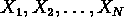of a random variable X, the order statistics are obtained by sorting the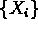in ascending order. This produces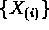satisfying: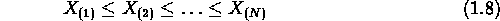The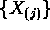are the order statistics of the N observations . An Order Statistic Filter (OSF) is a estimator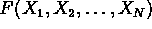of the mean of X which uses a linear combination of order statistics: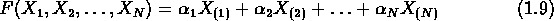Order Statistic Filters have long been known to statisticians as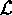-estimators, but were re-christened and applied to image processing problems by Bovik et. al. . Some common filters which fit the order statistic filter framework are:

• The linear average, which has coefficients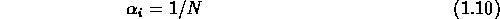• The median filter, which has coefficients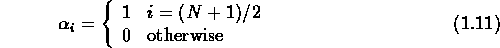For image processing applications, N is almost always odd, so the question of how to handle even values of N is avoided.

• The trimmed mean filter, which has coefficients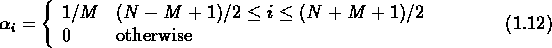For any distribution, one can determine the optimal coefficients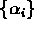by minimizing the criterion function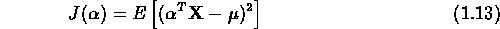where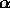is the vector of order statistic filter coefficients,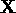is the vector of order statistics, and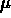is the mean of the random variable X. It turns out that the linear average is optimal for the Gaussian distribution. Table 1.1 gives OSF coefficients for Gaussian, Laplacian and Uniform noise, for the case N=9. Bovik  lists optimal coefficients for several other distributions.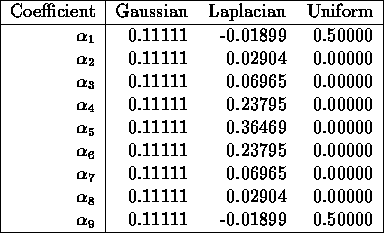Table 1.1: OSF filter coefficients for N=9

An aspect of order statistic filters which turns out to be important for Grid Filters is that they are piecewise linear. The filter partitions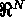into N! regions of the form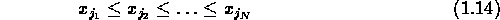where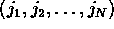is a permutation of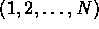. Over each of these regions, the filter output is a linear function (1.9).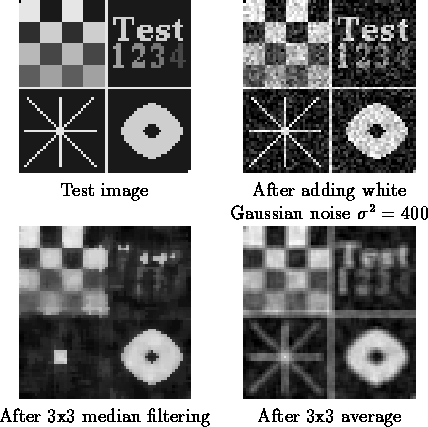Figure 1.3: Effect of local averages and median filters on fine details

To apply an order statistic filter to an image, one typically uses 3x3, 5x5 or 7x7 windows. For non-Gaussian noise, the optimal OSF is superior to taking a local average for flat regions. The main problem with such filters is the underlying stationarity assumption: the derivation of the OSF assumes that X is a stationary point process, an assumption which is grossly violated if there is an edge, line, or other strong signal activity in the window. Figure 1.3 illustrates this for a test image degraded by additive white Gaussian noise with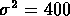. The median filter (lower left) preserves edges (the checkerboard and the bagel), but wipes out fine details (the text and lines). The 3x3 average, which is the optimal OSF for Gaussian noise, blurs too much.

These limitations motivated the development of the Adaptive Trimmed Mean Filter , which makes the assumption that the signal is smoothly varying within the local window. When the signal varies slowly within the window, the filter behaves like a trimmed mean. When an abrupt transition is detected, the filter behaves like a median, which preserves edges. Unfortunately, the median filter also destroys fine details, as illustrated in Figure 1.3. Another extension of OSFs to the nonstationary case, called a Permutation Filter or or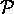-filter , has apparently not yet been tested on image restoration problems.Next: Lee's local statistics filter Up: Literature review Previous: Literature review

Todd Veldhuizen
Fri Jan 16 15:16:31 EST 1998